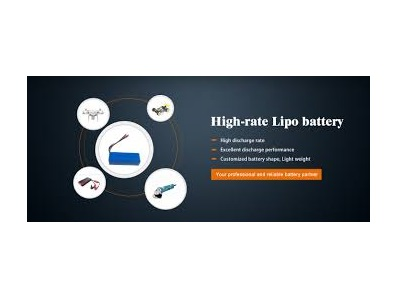# How to determine which "rate" you should use for your LiPo Battery ?If you’re new to LiPo batteries, you may be unfamiliar with how to safely set the charge rate for your battery. The battery manufacturer will tell you what the maximum “Charge Rate” of the battery is. This information is different than the “C-Rating” which is usually expressed as a number on the front of the pack (20C, 50C, etc).

If you are unsure of your batteries charge rate, it is safe to charge the pack at a 1C rate. To determine this number, take the mAh rating of your pack and divide it by 1000.

Example 1: If your battery is a 5000mAh pack, your 1C charge rate is 5A. (5000÷1000=5)

Example 2: If your battery is 2200mAh your 1C charge rate is 2.2A. (2200÷1000=2.2)

Example 3: If your battery is a 250mAh pack, your 1C rate would be .3. (250÷1000=.25 it’s okay to round up)

If your batteries allow for a higher charge rate (1C-5C is the general range) simply take the numbers you came to calculating your 1C rate and multiply it by that factor. So let’s use the above 3 examples.

Example 1: Our first example was a 5000mAh pack, which gives us a 5A charge rate at 1C.             A 2C charge rate would be 10A, a 3C charge rate would be 15A and a 5C charge rate would be 25A.

Example 2: 2200mAh 1C charge rate = 2.2A
2C charge rate= 4.4A

3C charge rate= 6.6A

5C charge rate= 11A
Example 2: 250mAh 1C charge rate = .3A

2C charge rate = .5A
3C charge rate = .8A

5C charge rate = 1.3A

You should now be confident when it comes to setting the “AMP” rate when charging LiPo batteries. Always double check that your voltage is set to the same one listed on your battery label and you’ll be out at the track or up in the air in no time!

Benzoenergy.com Provide high quality High rate battery.